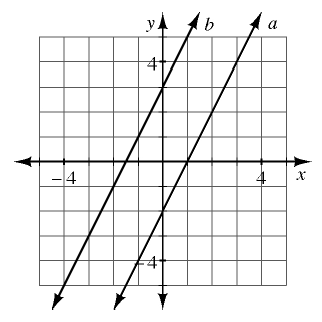### Home > CC3MN > Chapter 7 > Lesson 7.2.3 > Problem7-53

7-53.

When Yoshi graphed the lines $y=2x+3$ and $y=2x−2$, she got the graph shown at right.

1. One of the lines at right matches the equation $y=2x+3$, and the other matches $y=2x−2$. Which line matches which equation?

Look at the $y$-intercepts, otherwise known as the $b$ in
$y=mx+b$, to decide which line goes with which equation.

line $a$: $y=2x−2$, line $b$: $y=2x+3$

2. Yoshi wants to add the line $y=2x+1$ to her graph. Predict where it would lie and sketch a graph to show its position. Justify your prediction.

The slope of the line in part (c) is the opposite of the slope of the line in part (b). The $y$-intercepts are the same.

3. Where would the line $y=−2x+1$ lie? Again, justify your prediction and add the graph of this line to your graph.Use the eTool below to graph the line by completing the table.
Click the link at right for the full version of the eTool:  7-53 HW eTool